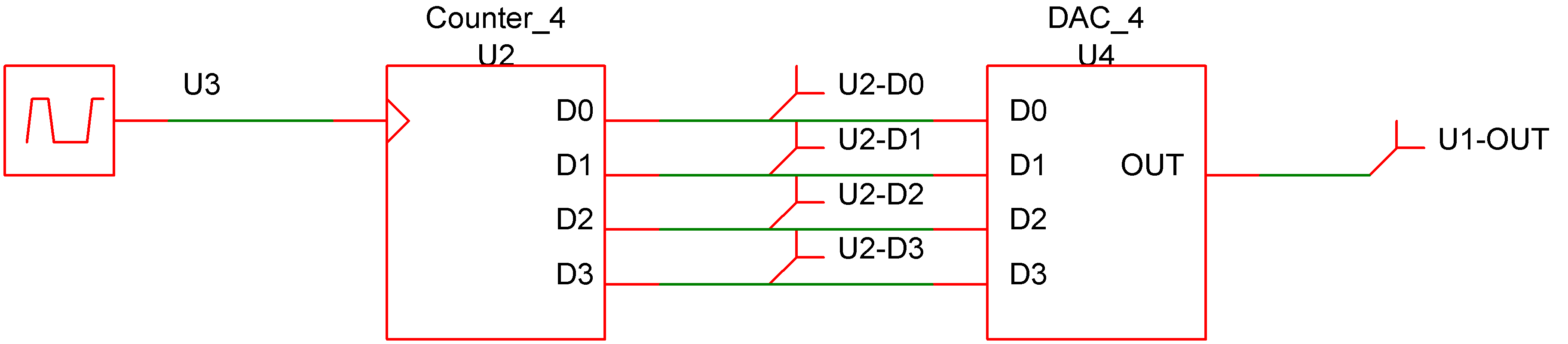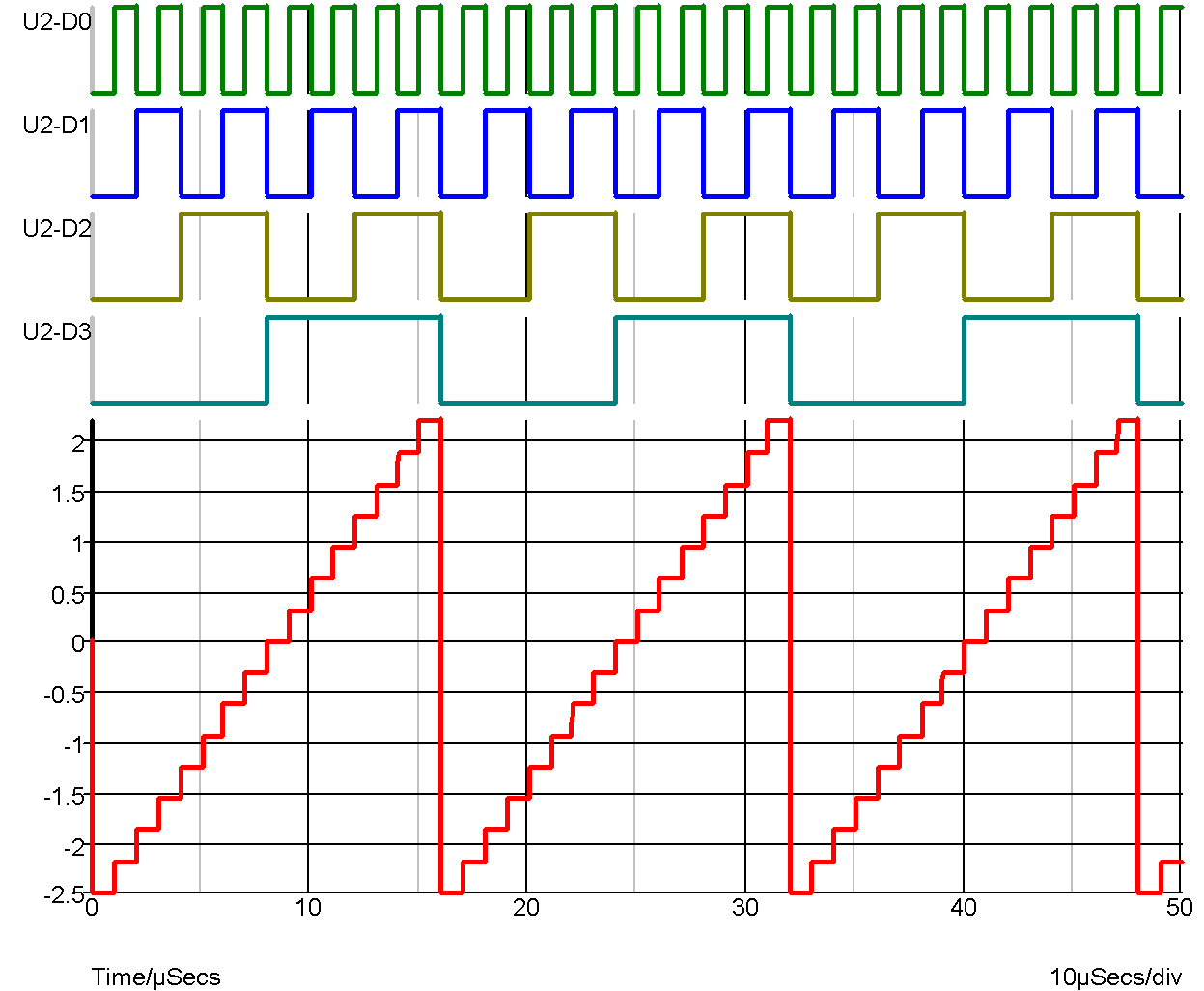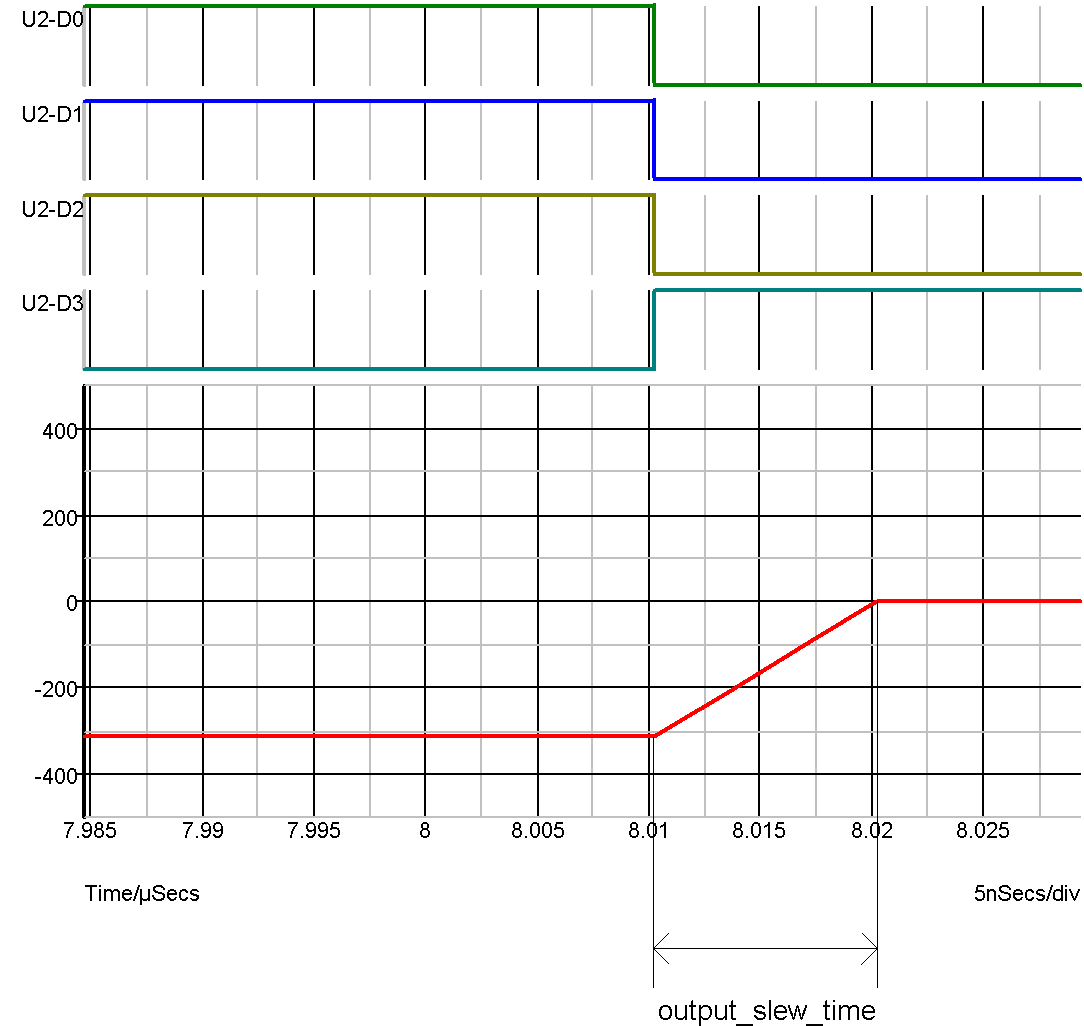# Digital-Analog Converter

In this topic:

## Netlist entry

Axxxx [ digital_in_0 digital_in_1 .. digital_in_n ]
+ analog_out model_name

## Connection details

 Name Description Flow Type Allowed types Vector bounds digital_in Data output in d d 1 - 32 analog_out Analog output out v v, vd, i, id n/a

## Model format

.MODEL model_name da_converter parameters

## Model parameters

 Name Description Type Default Limits output_offset Offset voltage real 0 none output_range Input signal range real 1 none twos_complement Use 2's complement input. (Default is offset binary) boolean FALSE none output_slew_time Output slew time real 10nS $1\text{e}^{-12} - \infty$ in_family Input logic family string UNIV none input_load Input load real 1pF $0 - \infty$ sink_current Input sink current real 0 none source_current Input source current real 0 none

## Device Operation

This device is a 1-32 bit digital to analog converter. Its operation is illustrated by the following diagrams.DAC WaveformsDAC waveforms expanded to show output slew

The device illustrated above has the following model definition:

.model DAC_4 da_converter
+ output_slew_time 1e-08
+ output_range 5
+ output_offset 0

In offset binary mode the D-A converter produce an output voltage equal to:

-OUTPUT_RANGE/2 + OUTPUT_OFFSET + code * OUTPUT_RANGE/2 n

where n is the number of bits and code is the digital input code represented as an unsigned number between 0 and $2^{n}-1$.

In 2's complement mode the output is:

OUTPUT_OFFSET + code * OUTPUT_RANGE/2 n

where n is the number of bits and code is the digital input code represented as a signed number between $-2^{n/2}$ and $2^{n/2}-1$.

Whenever the input code changes, the output is set on a trajectory to reach the target value in the time specified by OUTPUT_SLEW_TIME. UNKNOWN states are ignored. That is the input will be assumed to be at the most recent known state.